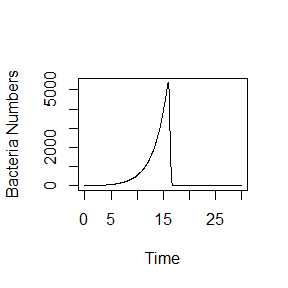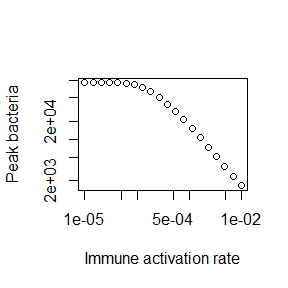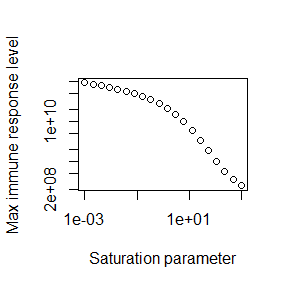# DSAIRM Package Tutorial

## Summary

Dynamical Systems Approaches to Immune Response Modeling (DSAIRM) is an R package that allows individuals to explore and learn about dynamical systems modeling of within-host infection and immune response dynamics, by interacting with models through a graphical user interface. It is not necessary - though possible - to read or write computer code.

The idea behind the specific structure of the package is that it provides a gentle introduction to immune response modeling that starts with a graphical user interface and takes students slowly to more advanced levels.

The different use cases for the package are described below.

## Package description

The package consists of several simulations/apps that allow for the simulation and exploration of different topics in within-host infection and immune response dynamics.

The underlying models are written as compartmental dynamical models, either deterministic using differential equations (deSolve package) or stochastic using a Gillespie-type approach (adaptivetau package). A graphical user interface is wrapped around each simulation/app. The graphical user interfaces are written using the functionality of the R Shiny package. This allows exploration of models without the need to write any code. At the same time, the package is structured in a modular way that should allow those interested in the actual models and learning R coding to easily move from one stage to another.

Each app is meant to be fully self-explanatory and contains a description of the model, a list of tasks the user could try, and information on further details and readings.

## Intended audience and goal of the package

The main audience are individuals who want to learn how mechanistic, dynamical systems models can be used to study within-host infection dynamics. While the ideal use of this package is likely as component of a class on this topic, self-learning might also be possible. Each app contains a fair amount of documentation and description of the model. By reading the model description, doing the tasks and probably also reading some of the papers in the future information section, it might be possible to teach yourself the beginnings of within-host modeling on your own.

## Installing the package

The package can be installed from CRAN or Github. See the documentation on the package page for more details.

Package installation is a one-time process, unless R itself is being upgraded/reinstalled. Note that the package depends on other packages, which will also be installed as needed.

## Using the package

The following sections describe the main envisioned ways the content in this R package can be used and extended. The idea is that everyone starts at level 1, and then, based on needs and interests, can decide to move on to the next level.

### Level 1: Interactive use through the graphical user interface

The interactive exploration of the models and infectious disease concepts through the graphical user interface is the main intended use of this package. The steps to get there are simple.

#### Load and run the package

Every time a new R/Rstudio session is started, the package needs to be loaded:

library('DSAIRM')

followed by starting the main menu for the package:

dsairmmenu()

This will bring up a graphical menu from which one can select each Shiny app. Each app contains the information needed to understand the underlying model, and has a list of (non exhaustive) suggested tasks to learn about the topic covered by the app. After exploring an app, the user returns to the main menu and eventually exits the main menu and closes the R session. No code needs to be written at this level of exploration and learning.

### Level 2: Directly interacting with the simulation functions

Once a user is comfortable interacting with the models and has a good understanding of the concepts covered by the different apps, it is possible, without too much effort, to interact with the simulation models directly. This provides more flexibility at the cost of writing a limited amount of code. To facilitate direct interaction with the models, each app is structured in such a way that the underlying simulation can be accessed without going through the graphical interface. The Further Information tab inside each app provides the name of the corresponding underlying function(s) and how to call their help files to get information on their use.

Consider as example the first app, called “Basic Bacteria Model”. This model has 2 underlying simulator functions, one that runs the discrete-time model called simulate_basicbacteria_discrete and one that runs the continuous, differential equation model called simulate_basicbacteria_ode. A user interested in the latter can learn about the inputs and outputs of the function by looking at its documentation. This can be achieved by by typing the following into the R console (make sure the DSAIRM package is loaded):

help('simulate_basicbacteria_ode')

The help file explains that one can run the simulation by specifying initial number of bacteria and immune response strength, the different model parameters, as well as some time values. Unless explicitly specified, the models do not have inherent time units. Instead, those are set by the user based on time unit choices for parameters. Each model input has some default, which is used if one calls the fuction without any additional information. One can modify those default settings. For instance, the following call to the simulator sets the rate at which bacteria grow, g, and the rate at which the immune response is induced and grows, r, to values different than the defaults, while using the default values for the remainder (this is equivalent to setting different inputs through the graphical interface in level 1):

result <- simulate_basicbacteria_ode(g = 0.5, r = 0.002)

Calling the simulation function executes the underlying model. For this example, the simulation function produces and returns time-series for the dynamics of each of the variables that are tracked. Those returned results can be further processed. As example, a basic plot of bacteria as function of time can be produced with this line of code:

plot(result$ts[,"time"],result$ts[,"B"],xlab='Time',ylab='Bacteria Numbers',type='l')Not all simulation functions return time series. For instance the function underlying the uncertainty and sensitivity app returns specific outcomes (maximum and final state of variables) for different parameter values. In every instance, the documentation for the function explains what is returned, so a user can take the returned results and further process them.

The ability to call the simulation functions without using the graphical interface allows for more flexibilty in exploring the models. For instance if a user wanted to explore the behavior of a model systematically for different values of a given parameter, this would need to be done manually through the graphical interface. Calling the function directly allows one to automate this. As an illustration, assume a user wanted to know how the peak bacteria load changes with changes in the immune response activation rate ,r, (while keeping all other parameters at some specified fixed values). The following lines of code show how this can be achieved. A loop is run over different r values, for each r value the simulation is run and the peak of the bacteria is recorded. At the end, peak of bacteria as function of immune activation rate is plotted:

#values for immune activation rate, r, for which to run the simulation
rvec = 10^seq(-5,-2,length=20)
#this variable will hold the peak values for each r
Bpeak = rep(0,length(rvec))
for (n in 1:length(rvec))
{
#run the simulation model for different values of r
#for simplicity, all other inputs are kept at their defaults
result <- simulate_basicbacteria_ode(r = rvec[n])
#record max number of bacteria for each value of r
Bpeak[n] <- max(result$ts[,"B"]) } #plot final result plot(rvec,Bpeak,type='p',xlab='Immune activation rate',ylab='Peak bacteria',log='xy')This simple example shows how a user can add just a few lines of extra code to the existing simulator functions and thereby explore scenarios much more flexibly than would be possible through the graphical interface. ### Level 3: Modifying the simulation functions While level 2 provides the user with a fair amount of flexibility and the ability to investigate the models and ask questions that would be hard or impossible going through the graphical interface, there is still one major constraint. Namely this approach only allows use and analysis of existing DSAIRM simulation models. This constraint can be overcome by directly modifiying and customizing the underlying simulation functions. As was true for moving from level 1 to 2, this move from level 2 to what I call level 3 provides further (almost unlimited) flexibility at the cost of having to write increasingly more R code. To make modifying the existing functions easy, copies of all simulator functions can be downloaded directly from the main menu. The code for each simulator function is (hopefully) well documented. Some basic to intermediate level of R coding experience is likely required to successfully modify the functions. In addition to modifying the simulator function of interest, a user will likely also have to write some additional code to interact with their modified function (as described in level 2). The following provides an illustrative example of this approach. Assume that a user wants to modify the simple bacteria ODE model. The code for this model is in simulate_basicbacteria_ode.R. (To make things easy, the name of a .R file containing the code and the name of the function itself are the same.) After finding the file, making a copy and renaming it (called mysimulator.R here), the user can make modifications. Let’s say the user wants to study a specific system for which this simple model is not completley suitable. Instead, for their their system, it is biologically more reasonable to assume that the immune system growth term includes saturation at some maximum rate when bacteria numbers are high. This can be accomplished by a change of the term rBI to rBI/(B+s). (See the documentation for this app for an explanation of each model term and corresponding equation). This change leads to a growth at rate rB proportional to the number of bacteria if B is smaller than some threshold parameter s, and turns into a growth at fixed maximum rate r, independent of bacteria numbers, if B is larger than s. The following modifications to the code will accomplish this: old: simulate_basicbacteria_ode <- function(B = 10, I = 1, g = 1, Bmax = 1e+05, dB = 0.1, k = 1e-06, r = 0.001, dI = 1, tstart = 0, tfinal = 30, dt = 0.05) new: mysimulator <- function(B = 10, I = 1, g = 1, Bmax = 1e+05, dB = 0.1, k = 1e-06, r=1e3, dI=1, tstart = 0, tfinal = 30, dt = 0.05, s=1E3) Note the changed default value for r to ensure the immune response is sufficiently triggered. old: pars = c(g=g,Bmax=Bmax,dB=dB,k=k,r=r,dI=dI) new: pars = c(g=g,Bmax=Bmax,dB=dB,k=k,r=r,dI=dI,s=s) old: dBdt = g*B*(1-B/Bmax) - dB*B - k*B*I dIdt = r*B*I - dI*I new: dBdt = g*B*(1-B/Bmax) - dB*B - k*B*I dIdt = r*B*I/(s+B) - dI*I With these changes made, it is now possible to use the new mysimulator function to ask specific questions regarding this model, and thus the underlying biological system of interest for which this model is a - hopefully useful - approximation. For instance one can explore how different values of the saturation parameter s impact the maximum level of the immune response. This requires a slight modification of the code shown above in Level 2 as follows: #initialize the new function #it needs to be in the same directory as this code source('mysimulator.R') #values of saturation parameter to explore svec = 10^seq(-3,3,length=20) #this will record the maximum immune response level Imax = rep(0,length(svec)) for (n in 1:length(svec)) { #run the simulation model for different values of s #for simplicity, all other inputs are kept at their defaults result <- mysimulator(s = svec[n]) #record max immune response for each value of s Imax[n] <- max(result$ts[,"I"])
}
plot(svec,Imax,type='p',xlab='Saturation parameter',ylab='Max immune response level',log='xy')Using one of the provided simulator functions as starting point and modifying it is likely easier than having to write a new model completely from scratch. Eventually, as a user aquires more coding experience, they are essentially only limited by what can be accomplished using the R programming language and their ability and interest in writing their own customized code.

## Further information

For users who want to engage further with DSAIRM, contributions are very welcome. It is quite likely that there are still bugs and typos in the package and its documentation. Users are encouraged to submit bug reports, feature requests, or any other feedback, preferably through the package’s Github site. The package is built in a way that makes it hopefully easy for others to contribute new apps. To that end, the package contains a subfolder called docsfordevelopers, which provides information on how the apps are structured and how to add new ones. This documentation file, documentation.md, provides further information. The information provided is meant to be detailed and complete enough to allow fairly easy development and contribution of new apps (or other enhancements) to the package. For any further questions, feel free to contact me via email or Github.

Also note that there is a ‘companion’ package to this one, called Dynamical Systems Approaches for Infectious Disease Epidemiology (DSAIDE). It focuses on models for infectious disease epidemiology (the population level). It has the same structure as DSAIRM. See the DSAIDE site for more information.